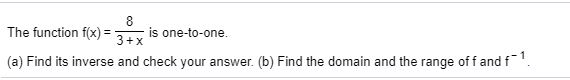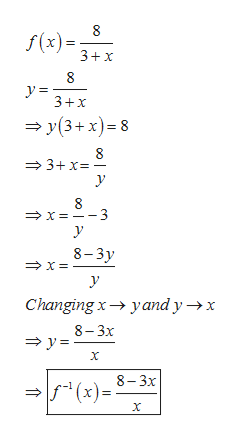# The function f(x) =is one-to-one.(a) Find its inverse and check your answer. (b) Find the domain and the range of f and f.

Question
12 viewshelp_outlineImage TranscriptioncloseThe function f(x) = is one-to-one. (a) Find its inverse and check your answer. (b) Find the domain and the range of f and f. fullscreen
check_circle

Step 1

F(x)= 8/(3+x)

For the given function

2. Find the domain and range of  f and f-1
Step 2

Inverse of the functionhelp_outlineImage Transcriptionclosef(x) = 3+x y = 3+x = y(3+ x)= 8 = 3+x=- →X = --3 8-3y Changing x→ yand y →x 8- 3x = y =- (x) = 8-3x (x)=: 00 fullscreen
Step 3

Checking the inverse...

### Want to see the full answer?

See Solution

#### Want to see this answer and more?

Solutions are written by subject experts who are available 24/7. Questions are typically answered within 1 hour.*

See Solution
*Response times may vary by subject and question.
Tagged in

### Other